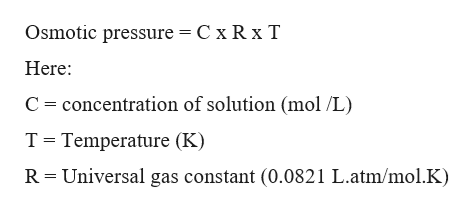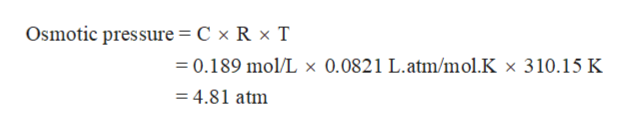# Calculate the osmotic pressure of a 0.189 M aqueous solution of sucrose, C12H22O11, at 37 °C.

Question
958 views

Calculate the osmotic pressure of a 0.189 M aqueous solution of sucrose, C12H22O11, at 37 °C.

check_circle

Step 1

Colligative properties are the properties which depend on the number of atoms or molecules or ions or particles present in the solution. Some common colligative properties are depression in freezing point, elevation in boiling point, osmotic pressure and relative lowering in vapor pressure.

Step 2

Osmotic pressure is a colligative property that can be calculated with the help of concentration of solution. The relation between osmotic pressure and concentration of solution can be written as:help_outlineImage TranscriptioncloseOsmotic pressure = C x R x T Here concentration of solution (mol /L) C T Temperature (K) Universal gas constant (0.0821 L.atm/mol.K) R fullscreen
Step 3

Calculate osmotic pressure for the given concentration of solution.

C = 0.189 M

T = 37 °C =...help_outlineImage TranscriptioncloseOsmotic pressure = C x R x T 0.189 mol/L x 0.0821 L.atm/mol.K x 310.15 K = 4.81 atm fullscreen

### Want to see the full answer?

See Solution

#### Want to see this answer and more?

Solutions are written by subject experts who are available 24/7. Questions are typically answered within 1 hour.*

See Solution
*Response times may vary by subject and question.
Tagged in